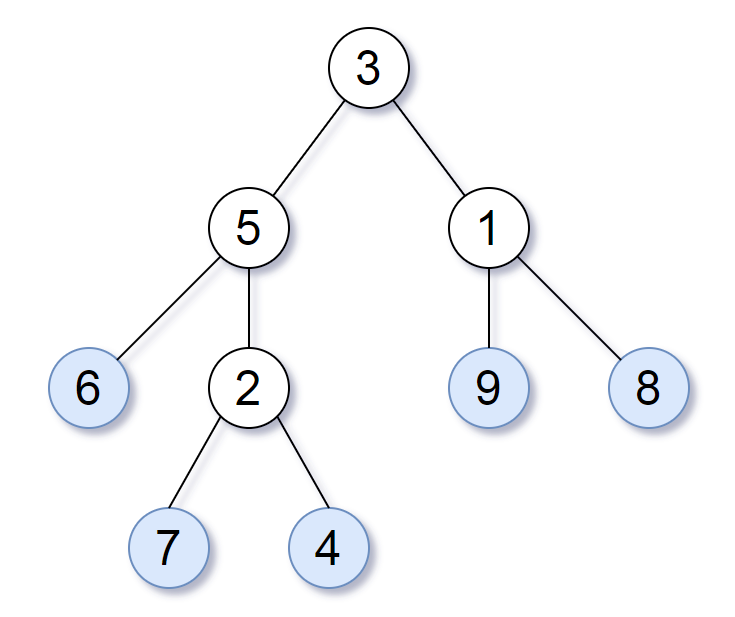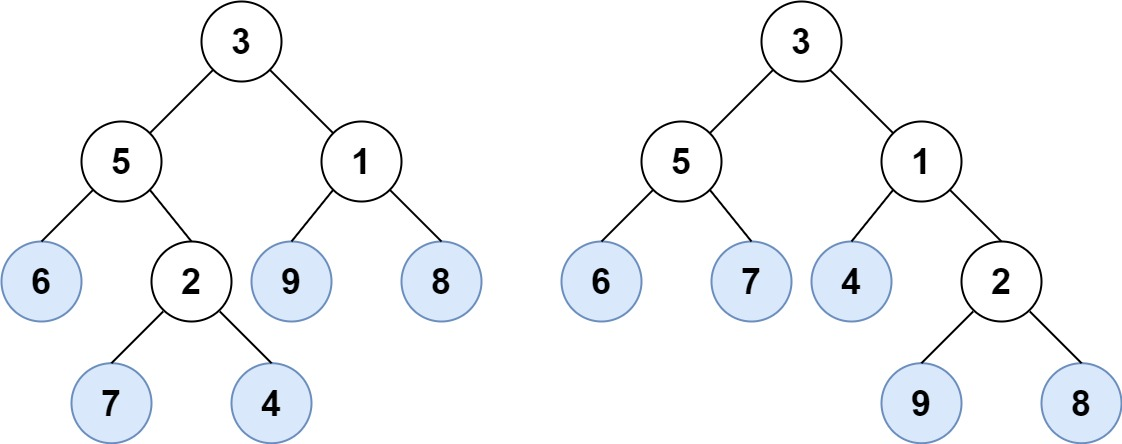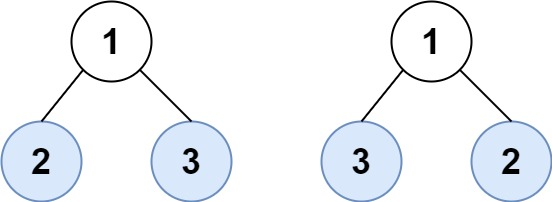# LeetCode: 872. Leaf-Similar Trees

## 题目¶

Consider all the leaves of a binary tree, from left to right order, the values of those leaves form a leaf value sequence.For example, in the given tree above, the leaf value sequence is (6, 7, 4, 9, 8).

Two binary trees are considered leaf-similar if their leaf value sequence is the same.

Return true if and only if the two given trees with head nodes root1 and root2 are leaf-similar.

Example 1:```Input: root1 = [3,5,1,6,2,9,8,null,null,7,4], root2 = [3,5,1,6,7,4,2,null,null,null,null,null,null,9,8]
Output: true
```

Example 2:

```Input: root1 = , root2 = 
Output: true
```

Example 3:

```Input: root1 = , root2 = 
Output: false
```

Example 4:

```Input: root1 = [1,2], root2 = [2,2]
Output: true
```

Example 5:```Input: root1 = [1,2,3], root2 = [1,3,2]
Output: false
```

Constraints:

• The number of nodes in each tree will be in the range [1, 200].
• Both of the given trees will have values in the range [0, 200].

## 解法¶

```# Definition for a binary tree node.
# class TreeNode(object):
#     def __init__(self, val=0, left=None, right=None):
#         self.val = val
#         self.left = left
#         self.right = right
class Solution(object):
def leafSimilar(self, root1, root2):
leafs_1 = self.collect_leafs(root1, [])
leafs_2 = self.collect_leafs(root2, [])
return leafs_1 == leafs_2

def collect_leafs(self, root, values):
if root is None:
return values

if root.left is None and root.right is None:
values.append(root.val)

self.collect_leafs(root.left, values)
self.collect_leafs(root.right, values)

return values
```